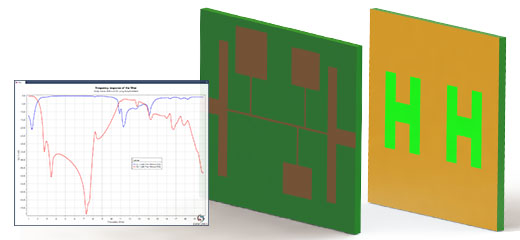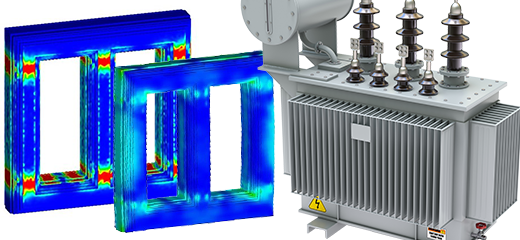# Which product should I use for my electronic design? EMS or HFWorks?

Low vs high frequency electromagnetics

Passive components Actuators, Solenoids & Electromechanical
By John Moz | 24/06/2021

Both EMS and HFWorks can readily be used to simulate electronic devices and circuits. It can truly be confusing to the user of which product to use.  In this article, we shall give some general guidelines on how to choose which one is more appropriate for your application.

EMS neglects the so-called Maxwell’s displacement current while HFWorks does not.  The displacement current is responsible for coupling the electric and magnetic fields which causes the propagation of electromagnetic fields.  But why does EMS neglect the displacement current? Because the operation frequency is “relatively” low which makes the wavelength much larger than the electrical size of the device.  As a rule of thumb, the following guidelines should be taken into consideration when choosing between EMS and HFWorks:

EMS is the product to use if:

• The wavelength is much larger than the device,
• The frequency of operation ranges from DC to a few hundred MHz's,
• The calculation force and torque are sought,
• The dielectric breakdown is of interest,
• To compute structural deformation due to electromagnetic force or heat.
• To calculate the saturation of the core,
• To study power integrity, power supply, and power management,
• To compute capacitance, inductance, and resistance,
• To study the skin and proximity effects,
• To calculate eddy currents,
• Motion is involved such as a motor or generator.

Use HFWorks if:

• The wavelength is smaller or comparable to the device,
• The frequency of operation ranges from few hundred MHz's to few hundred GHz’s,
• Far-field and antenna parameters are sought,
• To compute S-parameters, e.g., insertion loss and return loss.
• To calculate the ports characteristic impedance and propagation constant,
• TDR is of interest,
• Crosstalk and distortion are of interest,
• To examine the signal integrity of the device,
• To approximate the temperature rise of an electronic device due to dielectric conductor losses,
• Compute the resonant frequency and/or quality factor of a resonator.